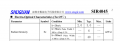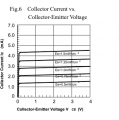# Simple photo diode tx distance

#### thedoc8

Joined Nov 28, 2012
162

#### Papabravo

Joined Feb 24, 2006
20,607
From the datasheet you can get a value for Radiant Intensity in milliwatts/steradian.In case you are unfamiliar with the unit known as the steradian it is a measure of solid angle. Considering a point source there are $$4\pi$$ steradians of solid angle in a sphere. Given one of the operating conditions for the forward current,$$I_F$$ , mentioned above, you can use the attenuation factor of $$1/r^2$$ to compute the intensity at any distance from the point source.

https://en.wikipedia.org/wiki/Inverse-square_law#:~:text=The intensity (or illuminance or irradiance) of light,one-quarter the energy (in the same time period).

To compute the maximum distance to a potential receiver you would need to know the sensitivity of that receiver. This might or might not be specified.

Here is the receiver sensitivity data:The median response would be a collector current of 3 mA with an irradiance of $$1.0\;mW/cm^2$$. That should give you what you need.

For example if you use 6.0 mW/sr case and a distance of 100 cm, you would have:

$$\cfrac{6.0 \text{ mW/sr}}{4\pi(100\text{ cm})^2}\;=\;47.75\text{ nW}/\text{cm}^2$$

It is doubtful this power level would provide much of a response, being several orders of magnitude too small.

Last edited:

#### thedoc8

Joined Nov 28, 2012
162
Thanks a mil, I knew the info was their, just to lacking in gray mater to figure it out. I will have at now with your info. Thanks again

#### BobTPH

Joined Jun 5, 2013
8,108
If you want good distance you will need to use a modulated signal and discriminating receiver. The TSOP type IR receivers are very sensitive and very good at rejecting background noise.